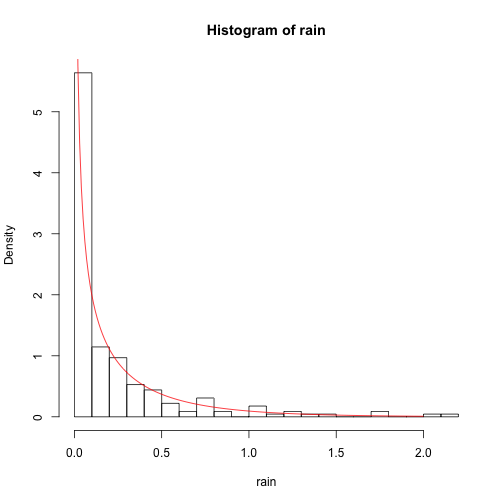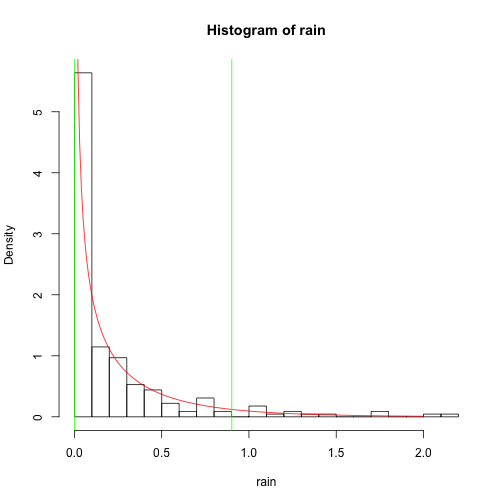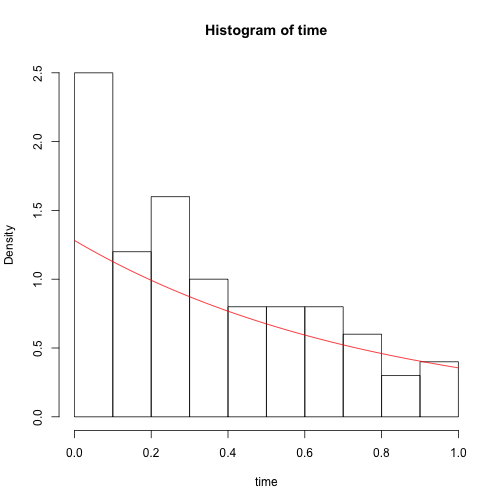## Exercise 1, Maximum likelihood; visualization

reading in the data and a bit of visualisation

``````illinois <- read.csv("illinois.csv", header = F)
rain <- illinois[, 1]
n <- 100

hist(illinois[, 1], breaks = "fd")
``````estimation of the parameters

``````etha <- seq(0.01, 1, 0.01)
lambda <- seq(0.01, 1, 0.01)

lik <- matrix(nrow = length(etha), ncol = length(lambda))
for (i in 1:length(etha)) {
for (j in 1:length(lambda)) {
lik[i, j] <- sum(dgamma(rain, shape = etha[i], scale = lambda[j], log = T))
}
}

which(lik == max(lik), arr.ind = TRUE)
``````
``````##      row col
## [1,]  44  51
``````
``````etha
``````
``````##  0.44
``````
``````lambda
``````
``````##  0.51
``````
``````
cor(lik[44, ], lik[, 51])
``````
``````##  0.8553
``````

So our shape parameter is estimated on 0.44 and our scale parameter on 0.51. We can nicely visualize our estimated density on a histogram of the data.

``````hist(rain, prob = T, breaks = "fd")
x <- seq(0, 2, 0.01)
lines(x, dgamma(x, shape = etha, scale = lambda), col = "red")
``````We can also see the contour of our density

``````library(lattice)
contour(lik[30:60, 30:80], xlab = "etha", ylab = "lambda")
``````## Exercise 2, Bootstrap

First we will look ath the 90% confidence interval assuming the data is from a gamma distribution

``````L1 <- qgamma(0.95, shape = etha, scale = lambda, lower.tail = T)
L1
``````
``````##  0.9019
``````
``````R1 <- qgamma(0.05, shape = etha, scale = lambda, lower.tail = T)
R1
``````
``````##  0.0004278
``````

so the 90% conf.int assuming the data is from a gamma distribution is between 0.0004278 and 0.9019067

``````hist(rain, prob = T, breaks = "fd")
x <- seq(0, 2, 0.01)
lines(x, dgamma(x, shape = etha, scale = lambda), col = "red")
abline(v = L1, col = "green")
abline(v = R1, col = "green")
``````The next confindence inteveral will be by non-parmetric bootstrap

``````L2.est <- R2.est <- numeric(10000)

for (i in 1:10000) {
s.rain <- sample(rain, 100, replace = T)
L2.est[i] <- sort(s.rain)
R2.est[i] <- sort(s.rain)
}

L2 <- mean(L2.est)
L2
``````
``````##  0.001324
``````
``````R2 <- mean(R2.est)
R2
``````
``````##  0.958
``````

so the 90% conf.int when doing a non-parametric bootstrap is between 0.0013201 and 0.957866

The difference in the two confidence intervals rely on a few things. Mainly the lower bound is much higher in the non-parametric case because when we sample from our date we will not get any lower values then 0.001, because this is our lowest value in the data.

## Exercise 3, Importance sampling

``````T2 <- numeric(1e+05)

for (i in 1:1e+05) {
x2 <- rnorm(10)
T2[i] <- max(x2)
}

sum(T2 > 4)
``````
``````##  35
``````

## Exercise 4, EM algorithm

``````survival <- read.csv("survival.csv", header = F)
time <- survival[, 1]
delta <- survival[, 2]
n2 <- 100
``````

Visualising the data

``````hist(time, breaks = "sturges")
``````Now we will construct an em algorithm, first the expectation part

``````E <- function(lambda, time, delta) {

lambda/(sum((delta * pgamma(time, 2, lambda)/pgamma(time, 1, lambda)) +
((1 - delta) * (1 - pgamma(time, 2, lambda))/(1 - pgamma(time, 1, lambda)))))

}
``````

The maximasastion part

``````M <- function(lambda0, iter = 10, delta, time) {

lambda.est <- numeric(iter)
for (i in 1:iter) {
lambda.m <- optim(lambda0, E, delta = delta, time = time, method = "Brent",
lower = 0.01, upper = 1)

lambda.est[i] <- lambda0 <- lambda.m\$par
}

lambda.est
}
``````

Now we can the algorithm, we will use a starting value of 0.5

``````
M(lambda0 = 0.5, iter = 10, delta = delta, time = time)
``````
``````##   0.01 0.01 0.01 0.01 0.01 0.01 0.01 0.01 0.01 0.01
``````

We can also use the liklihood of the observed data, which would look like this.

``````lambda2 <- seq(0, 1, 0.01)
lik2 <- numeric(length(lambda2))

for (j in 1:length(lambda2)) {
for (i in 1:n2) {
lik2[i] <- (1 - exp(-lambda2[j] * time[i]))^delta[i] * exp(-lambda2[j] *
time[i] * (1 - delta[i]))

}
}

which.max(lik2)
``````
``````##  79
``````
``````lambda2
``````
``````##  0.78
``````

By using the likelihood we find a lambda estimate of 0.78, we can visualize this in a histogram again

``````hist(time, breaks = "sturges", prob = T)
x3 <- seq(0, 1, 0.01)
lines(x3, dexp(x3, 1/0.78), col = "red")
``````